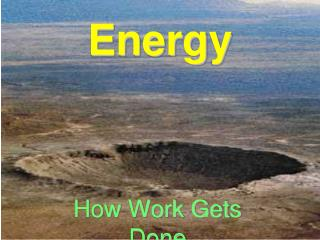Download PresentationEnergyEnergy - PowerPoint PPT Presentation

Download PresentationEnergy
An Image/Link below is provided (as is) to download presentation

Download Policy: Content on the Website is provided to you AS IS for your information and personal use and may not be sold / licensed / shared on other websites without getting consent from its author. While downloading, if for some reason you are not able to download a presentation, the publisher may have deleted the file from their server.

- - - - - - - - - - - - - - - - - - - - - - - - - - - E N D - - - - - - - - - - - - - - - - - - - - - - - - - - -
Presentation Transcript

1. Energy How Work Gets Done

2. What is Energy? Energyis the ability to cause change

3. Two General Types of Energy • Potential Energy • Kinetic Energy

4. What is Potential Energy? • Potential energy is the energy stored in an object. • Examples: • Chemical energy stored in batteries. • Mechanical energy stored in a compressed spring, or tight rubber band.

5. Gravitational Potential Energy • Gravitational potential energy is the stored energy of position. • A rock on top of a hill has more potential energy than the same rock at the bottom of the hill because the rock at the top has the added energy of being in a position where gravity can act on it longer. Weight (N) = mass (kg) x gravity (9.8 m/sec/sec) GPE = weight (N) x height (m)

6. What is Kinetic Energy?

7. Kinetic Energy • Kinetic energy is the energy of motion. • The faster something is moving the more kinetic energy it has. • KE =(m x v2) / 2

8. Forms of Energy • Mechanical Energy • Sound Energy • Chemical Energy • Thermal “Heat” Energy • Electromagnetic Energy • Light Energy. • Nuclear Energy

9. What is Mechanical Energy? • Mechanical energy is the motion of objects. • An example is the energy you use to pick up a book.

10. What is Sound Energy? • Sound energy is the vibration of particles in a solid, liquid, or gas. • Sound can not travel through empty space.

11. What is Electromagnetic Energy? • Electromagnetic energyis transmitted through space in the form of electromagnetic waves. • Electromagnetic energy is a combination of electrical and magnetic forces. • Visible light is a form of electromagnetic energy, as well as x-rays and microwaves. • The Sun releases large amounts of electromagnetic energy, some of which is absorbed by Earth.

12. What is Electrical Energy? • Electrical energy comes from the charges in atoms of matter. • Electrical energy is the force of opposite charges (“+” and “-”) attracting. • Lightning, static, and current are forms of electrical energy.

13. What is Magnetic Energy? • Magnetic energy comes from the organization of atoms in a material. • Magnetic energy is the force of opposite poles (north and south) attracting.

14. What is Nuclear Energy? Nuclear energy is the energy holding an atom together.

15. What is Chemical Energy? • Chemical energy is energy that is stored in the chemical composition of matter. • An example is the energy used by cells in your body comes from the chemical energy in the food you eat.

16. What is Thermal Energy? • Thermal energy, is the energy from the movement of molecules.

17. Thermal Energy – “Heat” • Heat is the transfer of thermal energy between objects. • Heat always flows from the warmer objects to the cooler objects. • Temperature is the measure of how fast the molecules of a substance are moving. The faster the molecules move, the higher the temperature.

18. Conservation of Energy • Energy can not be created nor destroyed. • Energy can be transferred from one form to another.

19. Energy Transfers • One form of energy can turn into another form. • An example is when a ball is thrown (mechanical energy) against a wall, some of this energy is converted into sound and heat so the ball does not bounce back as far. • Most energy that is “wasted” in a transfer is converted to heat energy.

20. Energy Transfers: Remote Car • Batteries (chemical to electrical) • Electrical (electrical to electrical) • Lights flash (electrical to light) • Motor turns (electrical to mechanical) • Motor makes noise (mechanical to sound) • Motor gets warm (mechanical to heat) • Wheels turn (mechanical to mechanical) • Friction on floor (mechanical to heat and sound)

21. Energy Transfers • Wind - up mouse • Jack - in - the - box